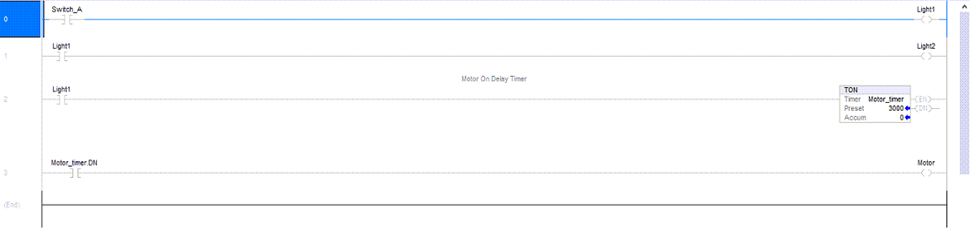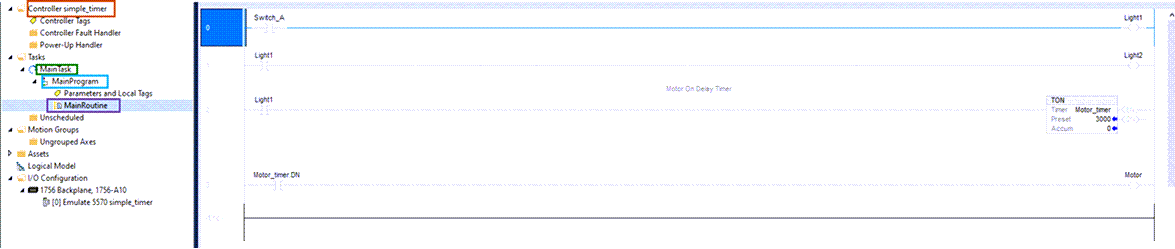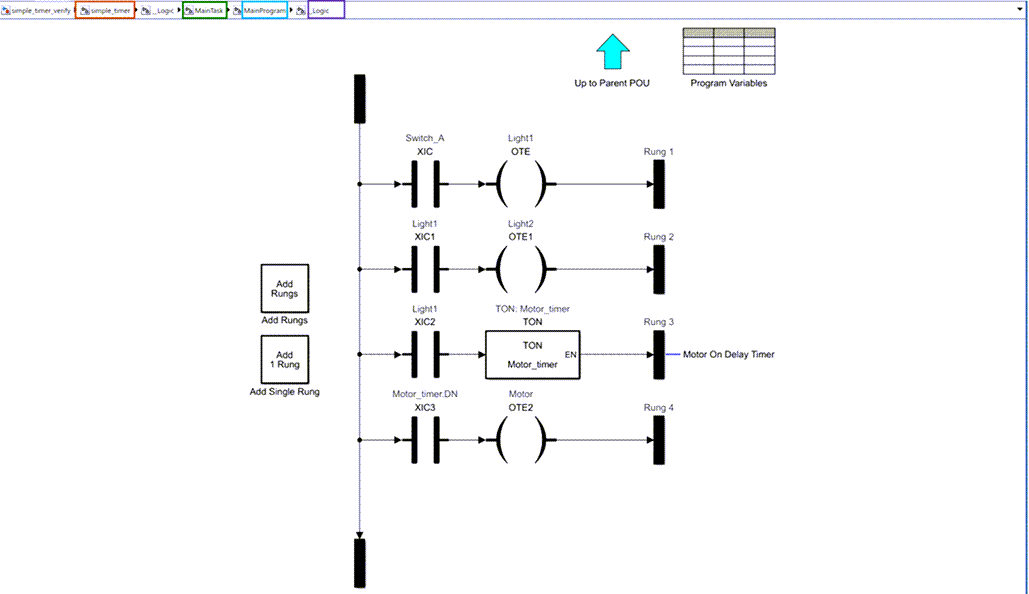## Syntax

``mdlname = plcimportladder(filename)``
``[mdlname,mdllib,genbusscript] = plcimportladder(filename,Name,Value)``

## Description

example

````mdlname = plcimportladder(filename)`generates a Simulink® representation of the ladder diagram in the L5X file created using Rockwell Automation® IDEs such as RSLogix™ 5000 and Studio 5000.```
``[mdlname,mdllib,genbusscript] = plcimportladder(filename,Name,Value)`generates a Simulink representation of the ladder diagram in the L5X file with properties specified using one or more `Name,Value` pair arguments.`

## Examples

collapse all

Simulate, test, and validate your `.L5X `ladder diagram files by importing your ladder diagram files into Simulink®. Use the `plcimportladder `function to import your ladder diagram files into Simulink. Simulink PLC Coder™ supports only import of ladder diagram files created by using Rockwell Automation® RSLogix 5000® and Studio 5000 ® integrated development environments (IDEs).

The ladder diagram in the `simple_timer.L5X `file controls a motor by using an input switch (`Switch_A)` and a timer (`Motor_timer). `This ladder diagram was created using the Studio 5000 IDE.`Light1, Light2, and Motor `are the outputs of this ladder diagram.

Before using the `plcimportladder `function to import your ladder diagram files into Simulink:

• Verify that your .`L5X `ladder diagram` `file has no errors by compiling the file in the Rockwell Automation IDE.

• Verify that the `.L5X `ladder diagram file uses blocks that are supported by Simulink PLC Coder. For a list of supported blocks at the MATLAB® command line, enter:

```plcladderlib ```

To import the `simple_timer.L5X `ladder diagram file into Simulink, use the `plcimportladder `function.

`plcimportladder('simple_timer.L5X');`

The ladder diagram is imported into Simulink and a `simple_timer.slx `file is created. The current folder also contains a `simple_timer_value.mat `file that loads the initial values for `Motor_timer` into the model data store memory. The data store memory also contains state information of elements of the ladder diagram. This state information is updated by the model during simulation.

• Imports rung comments. For example, rung two of `simple_timer.L5X` has the comment `Motor On Delay Timer. `This comment also appears in the Simulink model as well.

• Imports Add On Instruction (AOI) with mixed-order arguments, while preserving the order of the arguments. This order argument is preserved during ladder diagram code generation as well.

The `simple_timer.L5X ladder diagram file is located in `Controller simple_timer > MainTask > MainProgram > MainRoutine.The `simple_timer.slx `ladder diagram is located in `simple_timer > MainTask > MainProgram > _Logic. `This structure is similar to the structure in the Rockwell Automation IDE.To verify the imported ladder diagram:

• Connect a `Signal Editor `block to `Switch_A.`

• Connect a `Scope `block to the `Motor `and `Switch_A `signals.

• Open the `simple_timer_verify.slx `model.

• Open the `Scope `block and click the `Run` button.

```open_system("simple_timer_verify.slx") ```

This image shows the `Scope `block output for the model simulation. The `Motor (simple_timer) `output turns on three seconds after `Switch_A is turned on `and turns off as soon as `Switch_A `is turned off. This behavior is the expected behavior of the ladder diagram.## Input Arguments

collapse all

Specifies the name of the ladder file to read. Depending on the location of your file, you can either specify the name of the file or provide the full or relative path. name.

### Name-Value Arguments

Specify optional pairs of arguments as `Name1=Value1,...,NameN=ValueN`, where `Name` is the argument name and `Value` is the corresponding value. Name-value arguments must appear after other arguments, but the order of the pairs does not matter.

Before R2021a, use commas to separate each name and value, and enclose `Name` in quotes.

Example: `[mdlName,mdlLib,genBusScript] = plcimportladder('sampleLadder.L5X','OpenModel','on','TopAOI','sampleAOI')`

At the end of import process, the model is hidden by default. To keep the model open at the end of import, set the value of `OpenModel` to `on`.

Specify the function blocks that is to be imported. The software imports it into a ladder diagram 'runner' block.

## Output Arguments

collapse all

Specifies the name of the generated Simulink model.

Specifies the name of the generated Simulink model library.

Specifies the name of the generated bus script.

## Version History

Introduced in R2018a